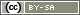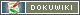[[id()]]

# id()

`string id(string category, integer question)`

`string id(string category, integer question, integer Item)`

`string id(string question identifier, integer Item)`

The function `id()` is generating a formally correct question- or item identifier out of single statements about the category, question and (optional) item. This question- or item identifier can be used e.g. in `value()` or `put()`. The function can be helpful, when the item identifier is specified as a number, like e.g. `1` instead of `01`.

• Category – Double-digit identifier of a category, e.g. `'AB`'
• Question – Numeric identifier of a question within a category, e.g.`1`, `'01`'
• Question identifier – Entire identifier of a question (incl. category), e.g. `'AB01`'
• Item – Numeric identifier of an item or an option within a question, e.g. `2`, `'02`'

## Return value

The function delivers the entire identifier of a question (e.g. `'AB01`') or an item, when the parameter Item was set (e.g. `'AB01_01`').

## Example

The following example lists options within a scale SK01, which were rated with a min. value of 3 from the participant (e.g. on a scale of 1 to 5). The evaluation should be shown in brackets. The evaluation is generated by `value()`. The therefore necessary variable-identifier (which matches the item-identifier) is generated with `id()`.

```// find items with evaluation 3+
\$items = getItems('SK01', 'min', 3);
// go through all items
foreach (\$items as \$item) {
// identify text
\$text = getItemtext('SK01', \$item);
// identify value
\$wert = value(id('SK01', \$item));
// show in questionnaire
html('<p>'.\$text.' ('.\$wert.')</p>);
}```